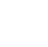Back to Chapter
Getting StartedBasic Syntax & DeclarationControl StatementsNo items found.
Function & EventsNo items found.
Array and its TypesNo items found.
OOP : Object Oriented ProgrammingNo items found.
Javascript StandardsNo items found.
HTML DOMNo items found.No items found.No items found.No items found.

# Arrow Function

Arrow Function is used to create functions in a cleaner way compared to regular functions. This Function is introduced in the ES6 version of JavaScript.

## Difference Between Normal Function and Arrow Function

1. Arrow Function is like the Shortcut for Creating Normal Function.
2. In Arrow Function code looks cleaner as compared to Normal Function.

## Syntax

We will Compare the syntax of both function Normal Function and Arrow Function

Normal Function :

``` function functionName(parameters){ //statements return variableName; } var returnedValue = functionName(parameters); ```

Arrow Funtion:

``` let returnedValue = (parameters) => { // statements return variableName; } ```

Here,

We have Created a variable with name returnedValue and parsing parameters in place of parameters and variableName is the Returned Value.

Lets See Some Examples To make it clear.

## Example

Lets Make a program that calculates the percentage of 5 Marks out of 100.

First in Normal Function :

``` function calcPercentage(m1,m2,m3,m4,m5){ let result = ((m1+m2+m3+m4+m5)/500) * 100; return result; } var result = calcPercentage(98,95,90,94,85); console.log(result); ```

Now do it with Arrow Function :

``` let myfunc = (m1,m2,m3,m4,m5) => ((m1+m2+m3+m4+m5)/500)*100 var result = myfunc(98,95,90,94,85); console.log(result); ```

Here,

We have created a let variable, and have Defined function in it.

We are sending 4 Variables m1, m2 , m3 , m4 , m5

Then, we have used formula to calculate the percentage : ((sum of all marks / Total Marks)*100)

The value of result will be printed in Console Tab.